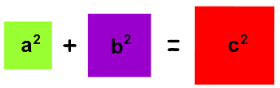Pythagorean TripletsA Pythagorean triplet is a set of three natural numbers, $a < b < c$, for which ${ a }^{ 2 }+{ b }^{ 2 }={ c }^{ 2 }$. For example, ${ 3 }^{ 2 }+{ 4 }^{ 2 }=9+16=25={ 5 }^{ 2 }$.

There exists exactly one Pythagorean triplet such that $a + b + c = 1000$. Find the value of $c$.

×